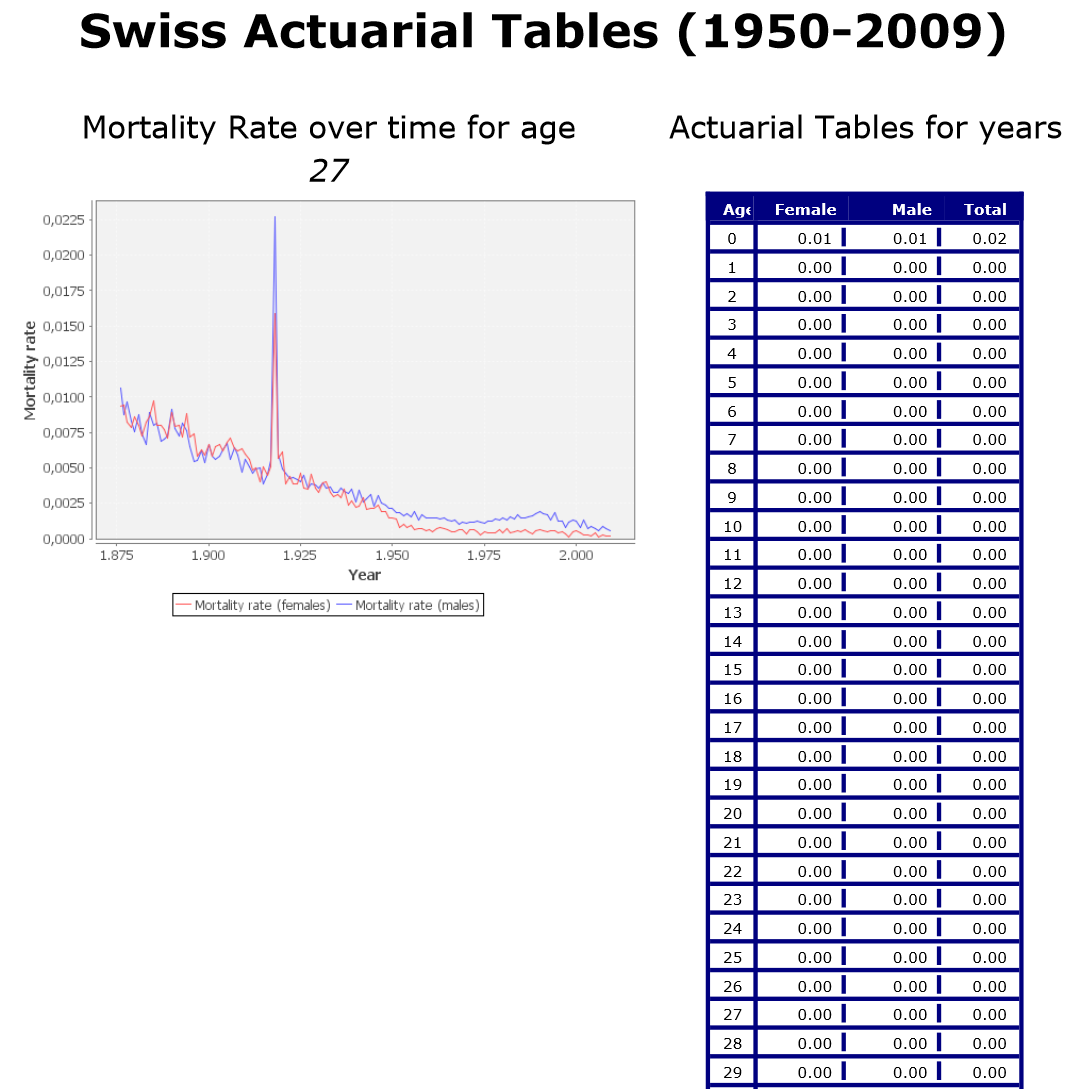# Actuarial Tables: Creation, Exploration, and Dynamic Report Generation

This workflow can be found on the KNIME EXAMPLES Server under 50_Applications/15_Swiss_Actuarial_Example/01_Swiss_Actuarial_Example

## Goal and Data Set

This use case shows how to generate actuarial tables with mortality rate and life expectancy for a given set of data.

The data sets used for this use case are publicly available and free of charge from the Human Mortality Database (HMD) at www.mortality.org. We used two files from the database:

1. DeathsSwitzerland.csv counts the number of deaths in Switzerland by age and gender for each year between 1876 and 2009;
2. ExposuresSwitzerland.csv counts the Swiss population by age and gender for each year between 1876 and 2009.

## Actuarial Figures Calculation

Based on the number of deaths and on the count of actual population for each age value and for each year, we can calculate the Mortality Rate and the LOG Mortality rate for male and female by age and year, as:

Mortality Rate (age, gender, year) = number of deaths (age, gender, year) / population count (age, gender, year)

LOG Mortality Rate (age, gender, year) = log10 (Mortality Rate)

However, actuarial figures have a predictive value only if calculated on a long enough time interval. We could use the whole 1876-2009 year range, but this would mix too different time periods with very different medical care techniques and plans. We then divided the whole time window in three ranges: 1876- 1900 for the medical ancient times, 1900- 1950 with the new medical discoveries, and 1950-2009 with modern medical care. The previous actuarial figures (mortality rate and log mortality rate) have then been calculated across each one of these three medical ages, replacing the single year with the year range in the formula above.

The example workflow, named “Swiss Actuarial Example”, implements two tasks:

• An interactive exploration of the mortality counts by age and gender for the three year ranges
• A report that selects a given age value, displays the mortality rate evolution over time (1876-2009) for the selected age, and shows the actuarial figures for all ages over the most recent year range 1950-2009.

## Interactive Exploration and Highlighting

The figure below shows the upper branch of the example workflow, displaying the interactive histograms of the number of deaths for a given age in a selected year range.

In order to associate a color to gender (red for female, blue for male, and green for total), the deaths and population counts are split for male, female, and total in the metanode named Metanode. Then a Numeric Binner node builds the three buckets: 1876-1900, 1900-1950, and 1950-2009. Finally, three Histogram (Interactive) nodes show the number of deaths (= aggregation column) by age (= binning column) for each year range. Average as aggregation method produces the average number of deaths for each age bin. Sum as aggregation method would sum all death numbers in each age bin.Below you can see the three histograms for the three year buckets: 1876-1900, 1900-1950, and 1950-2009.

Notice the progressive reduction in death count over the first year of life and the progressive increase for the latest age bins across the three histograms.

 Death Counts by Age in 1876-1900 Death Counts by Age in 1900-1950 Death Counts by Age in 1950-2009We also introduced an Interactive Table node to find specific groups of records. For example, it could be interesting to isolate the death counts of the few centenaries before 1900. By highlighting (Hilite -> Hilite Selected) the last bar in the 1876-1900 histogram and selecting the option Hilite -> Filter -> Show Hilited Only in the Interactive Table view, we get the table below with the full extract of death counts for ages older than 99.## Dynamic Report Generation

The lower branch of the workflow implements a few tasks:

• Calculates female and male mortality rate figures across 1876-2009 (“Actuarial Figures M/F” metanode)
• Caluclates the actuarial figures for all ages across 1950-2009 (“Actuarial Calculation year_Range” metanode)
• Selects an age value (“Actuarial Selection” metanode)
• Displays these mortality rates in a line chart image with the “Line Chart (JFreeChart)” node for the selected age value
• Builds a report with the actuarial table and the mortality rate line chart imageThe “Actuarial Calculation year_range” metanode specifically calculates the total number of deaths in each year range for each age value (GroupBy node), then calculates the Mortality Rate and the LOG Mortality Rate for each age with two Math Formula nodes (see figure below). Similarly the “Actuarial Figures M/F” metanode uses two Math Formula nodes for the mortality rates calculation: one for the male and one for the female population.Notice that the “Actuarial Selection” metanode contains a Value Selection Quickform node to select the age value. This Quickform node produces the age selection menu in the configuration window of the metanode, as shown below.The last figure shows the final report with the mortality rate trend for age 27 and the actuarial table for the most recent year range.* The link will open the workflow directly in KNIME Analytics Platform (requirements: Windows; KNIME Analytics Platform must be installed with the Installer version 3.2.0 or higher)